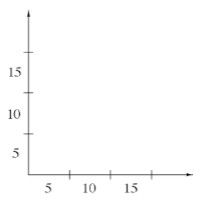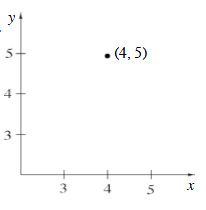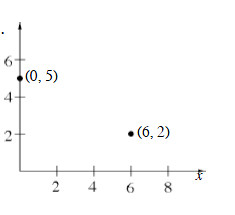### Home > MC1 > Chapter 7 > Lesson 7.1.2 > Problem7-26

7-26.

Some of the axes and graphs below have errors, and some do not. For each one, decide if it contains errors. If there are errors, redraw the graph correctly.1.This one is wrong. Think about how we indicate exactly where each value on the axes is represented.

1.This one is wrong too. Where is $0$ on the graph?
On each axis, the distance between $0$ and $3$ should be the same as the distance between $3$ and $6$.
How could you redraw this one correctly?

1.This one is correct!